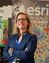# Comparable Graphs with ArcPy

878
6
02-02-2017 02:12 AMbyEsri Regular Contributor

I managed to create nice graphs saved to png (and svg or pdf). I have about 500 points for which I want to create this kind of graph. Now I wonder, if there is a way to take the minimum and maximum value of all these records to define the extend of my graph to make them comparable. E.g. each graph shows the temperature per depth but per point of measurement there is a difference in the temperature. In one point the temperature range might be 20-23 degrees, in another point 19-24. To make the graphs comparable it would be nice to have the option to define a minimum and maximum value that would be used for the definition of the axis.

Tags (3)
6 RepliesbyMVP Esteemed Contributor

Controlling the axes of the graph—Help | ArcGIS Desktop suggests you can control the axes range, but you would have to check to see if these values can persist between graphs.  MatPlotlib provides a much more controlled environment for batch processing of graphs (it is builtin to current version of arcmap).byMVP Frequent Contributor

ArcMap's graphing is okay and I have used it many times, but batching through arcpy I think would be quite difficult.

You may be able to set up a graph template and utilise that...

But I would have to second Dan Patterson

Matplotlib and matplotlib.pyplot is excellent and batching would be easy to set up. Lots of examples online..byEsri Regular Contributor

Thank you both for your comments. I am in fact using the Matplot library and now also managed to manipulate the axis in the desired way, thank you! Now I just have to figure out how to find the min and max values through all my files...byMVP Esteemed Contributor

RasterToNumPyArray—Help | ArcGIS Desktop the second example

you just need to cycle through the rasters and convert them to arrays, then get the min and max of the parsed list.  If you want to read the rasters one at a time, then you can just retain the current values and find the min and max as you go through.

assume the rasters came from using rastertonumpyarray as in the 2nd example

``````>>> a = np.arange(36).reshape(6,6)
>>> b = a[:]*0.5
>>> c = a[::-1]
>>> a
array([[ 0,  1,  2,  3,  4,  5],
[ 6,  7,  8,  9, 10, 11],
[12, 13, 14, 15, 16, 17],
[18, 19, 20, 21, 22, 23],
[24, 25, 26, 27, 28, 29],
[30, 31, 32, 33, 34, 35]])
>>> b
array([[ 0.000,  0.500,  1.000,  1.500,  2.000,  2.500],
[ 3.000,  3.500,  4.000,  4.500,  5.000,  5.500],
[ 6.000,  6.500,  7.000,  7.500,  8.000,  8.500],
[ 9.000,  9.500,  10.000,  10.500,  11.000,  11.500],
[ 12.000,  12.500,  13.000,  13.500,  14.000,  14.500],
[ 15.000,  15.500,  16.000,  16.500,  17.000,  17.500]])
>>> c
array([[30, 31, 32, 33, 34, 35],
[24, 25, 26, 27, 28, 29],
[18, 19, 20, 21, 22, 23],
[12, 13, 14, 15, 16, 17],
[ 6,  7,  8,  9, 10, 11],
[ 0,  1,  2,  3,  4,  5]])
>>> mins = np.min((a,b,c))
>>> maxs = np.max((a,b,c))
>>> mins
0.0
>>> maxs
35.0
‍‍‍‍‍‍‍‍‍‍‍‍‍‍‍‍‍‍‍‍‍‍‍‍‍‍‍‍‍‍``````byEsri Regular Contributor

Thank you! Very helpful. I don't have rasters but txt or dbf files but in theory that should work about the same. For the moment we decided to set the min and max manually.

In the meanwhile I managed to print 5 subplots (Temperature, pH, Conductivity, Pressure...)  in one figure, so I'm on a good way...byMVP Esteemed Contributor
``````>>> mn = np.random.randint(1,10, size=10)
>>> mx = np.random.randint(11,20, size=10)
>>> mn
array([7, 3, 3, 2, 4, 4, 9, 4, 9, 4])
>>> mx
array([12, 14, 12, 17, 11, 16, 16, 17, 12, 18])
>>> vals = list(zip(mn,mx))
>>> tbl = np.array(vals, dtype=[('min', '<i4'), ('max', '<i4')])
>>> tbl
array([(7, 12), (3, 14), (3, 12), (2, 17), (4, 11), (4, 16), (9, 16), (4, 17),
(9, 12), (4, 18)],
dtype=[('min', '<i4'), ('max', '<i4')])
>>> tbl_min = tbl['min'].min()
>>> tbl_max = tbl['max'].max()
>>> tbl_min, tbl_max
(2, 18)
‍‍‍‍‍‍‍‍‍‍‍‍‍‍‍‍‍‍‍‍‍‍‍‍‍‍‍‍‍‍‍‍‍``````

more homework TableToNumPyArray—Help | ArcGIS Desktop which will produce a structured array as shown in line 10-12.  ie the array will have named fields from which you can can get your values as in the examples from the subsequent lines